# Current Research – Superconductivity

## Nonlocal electrical transport measurements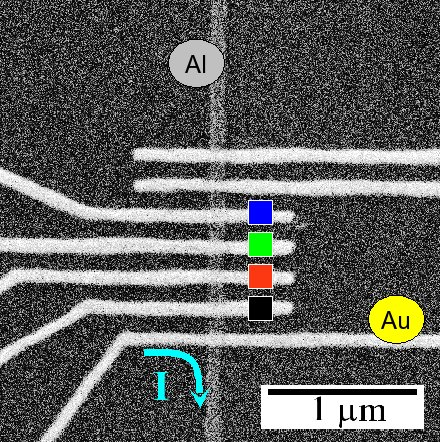Figure 1: Scanning electron micrograph of a hybrid superconducting/normal-metal device used to investigate nonlocal effects due to crossed Andreev reflection (CAR) and elastic cotunneling (EC).

On the microscopic level superconductivity is caused by the entangling of electrons into Cooper pairs. This naturally occurring pairing offers the opportunity to investigate quantum coherence and nonlocality in solid-state devices.

Our recent research has focused on the phenomenon of Crossed Andreev Reflection (CAR) wherein the the two electrons of a Cooper pair in a superconductor can be probed by spatially separated normal metal probes. As the effective size of a Cooper pair in a conventional superconductor such as Aluminum is a few hundred nanometers, the separate normal probes can be fabricated by conventional e-beam lithography.

Figure 1 shows an annotated scanning electron micrograph of a sample used to detect the nonlocal effect of entangled Cooper pairs.  It consists of an aluminum wire (vertical dark line) crossed by seven normal gold leads separated from each other by 200 nanometers. A current is sent from the bottom gold lead into the lower section of the aluminum wire and the other leads are used to measure the nonlocal resistance at different distances from the current path.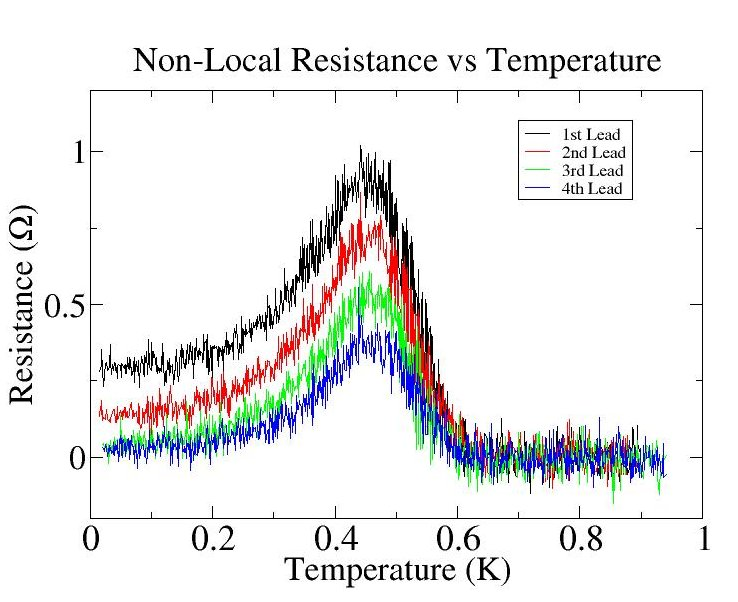Figure 2: Nonlocal resistance as a function of the temperature of the sample in Figure 1. The large peak in resistance observed at 0.6 K is due to charge imbalance, while the finite nonlocal resistance at base temperature for the first and second leads is due to a combination of CAR and EC.

Figure 2 shows the nonlocal resistance of the four closest leads as a function of temperature. Above the transition temperature of 0.6 K the aluminum is normal and there is no nonlocal signal, below the transition there is a large peak in the nonlocal resistance which decreases with distance. These peaks are not primarily due to coherent effects, but rather the nonequilibrium effect of Charge Imbalance (CI), the creation of an excess of “hole-like” quasiparticles in the superconductor. However, as the sample is cooled to close to zero temperature the quasiparticle creation is frozen out, and the remaining nonlocal signal is due to effects of coherently entangled electrons in which one electron is part of the current path and the other enters the nonlocal leads and to the related coherent effect of Elastic Cotunneling (EC). As the Cooper pairs only span a few hundred nanometers the nonlocal signal disappears by the third nonlocal lead.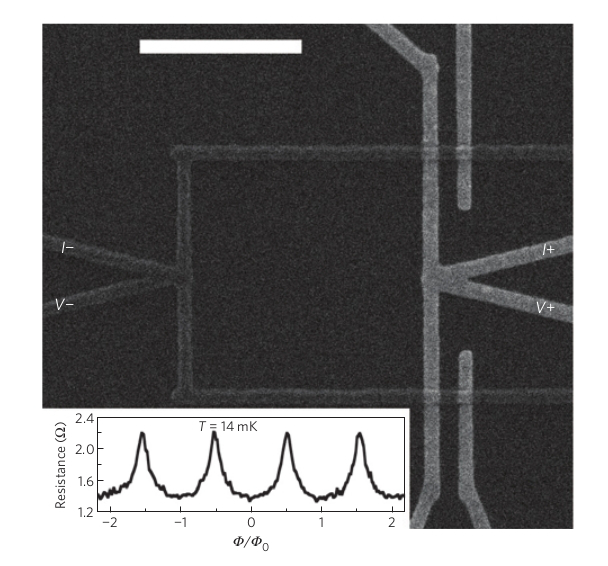Figure 3: Nonlocal measurements on an Andreev interferometer, a hybrid superconductor/normal-metal device where the phase of the carriers can be modulated by applying a magnetic flux through the area of the loop. The inset shows the modulation of the differential resistance with the applied flux, with a fundamental period of h/2e.

For other work on CAR and EC see investigations by the groups of Beckmann and Morpurgo.

One important aspect of nonlocal correlations such as EC and CAR is that they are “coherent” processes. The coherence of the nonlocal correlations can be verified by modulating the phase between two superconductors. Figure 3 shows a scanning electron micrograph of a sample where the aluminum wire is extended to form a loop with the gold wire. The inset of the figure is the differential resistance of the loop which shows the phase modulation of the sample by external magnetic field.

Figure 4 shows the nonlocal differential resistance of the Andreev interferometer of Figure 3 as a function of the applied flux.  The oscillations observed are periodic and antisymmetric in the applied flux, and are visible only in the presence of a finite dc current.  Note that the measurement is truly nonlocal, in that the dc and ac currents do not even have to be injected into the “sample.”  The oscillations arise from the nonequilibrium distribution induced by the dc current which induces a phase coherent quasiparticle current to be injected into the superconductor, which in turn results in a nonlocal voltage through EC/CAR.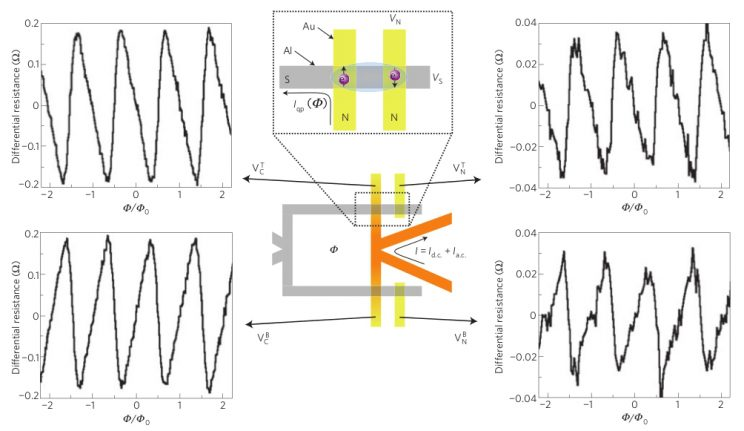Figure 4: Oscillations of the nonlocal resistance of an Andreev interferometer. The oscillations are antisymmetric with respect to the applied flux, and can only be seen if there is a finite dc current applied.

## Using Cross-Correlation Noise Measurements to Probe Quantum Coherence

For a three-terminal device with two normal-metal wires separated by a superconducting wire, it is interesting to know whether the electrons in the two normal-metal wires are in a quantum entangled state induced by the adjacent superconducting wire if the separation between them in the superconductor is small enough.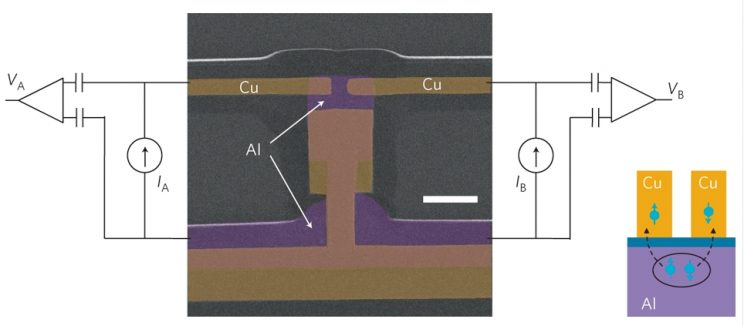Figure 5: Sample used to measure the cross-correlation noise from CAR and EC. Brown regions are copper, purple regions are superconducting aluminum, with a tunneling barrier between the two metals. The cross-correlation noise VA × VB is measured as a function of the bias currents IA and IB.

One possible microscopic process that can induce the entangled state is called Crossed Andreev Reflection (CAR), discussed above. The physical picture of CAR process can be thought of as follows: near the two normal-metal/superconducting interfaces, one  electron from each of the two normal-metal leads is converted simultaneously to (or is emitted from) a Cooper pair in the superconducting wire. Since the electrons disappear from the normal-metal wires at the same time, they are positively correlated and the currents flowing through the NS interfaces should also be positively correlated. One the other hand, it is also possible that the  electron can tunnel from one normal-metal wire to the other without being converted to Cooper pair, a process called Elastic Cotunneling (EC). In this case, due to the Pauli principle, electrons will tunnel one by one and this leads to negative correlation.

A simple transport measurement can not identify whether the correlation is positive or negative since it only gives the average value of the current. In contrast, a measurement of the current-current correlation is capable of separating the contribution from CAR and EC, thus determining whether or not there is an entangled state. The magnitude of the correlation is determined by the ratio of the separation distance between normal-metal wires over the superconducting coherence length.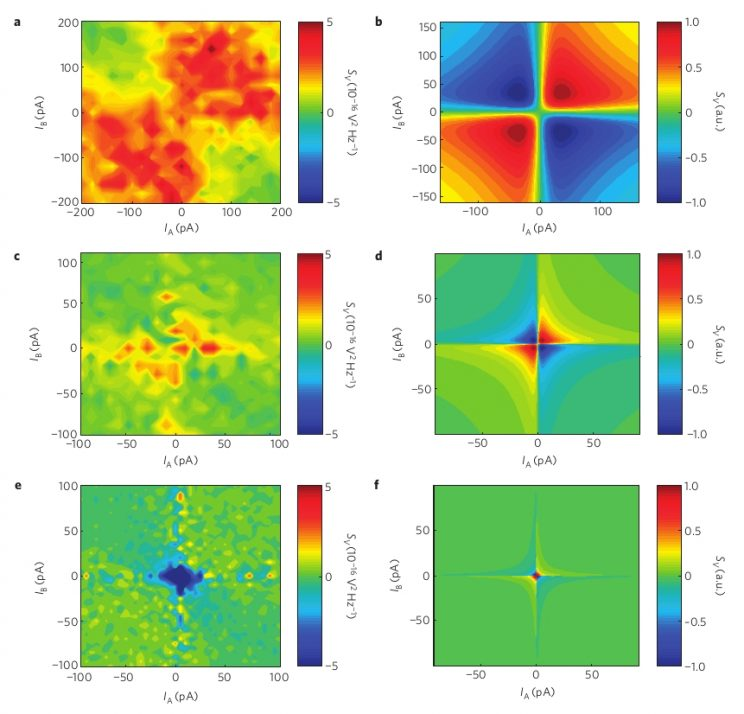Figure 6: Left panels: Cross-correlation noise data for the sample of Figure 5 for 0.4 K (top), 0.3 K (middle) and 0.25 K (bottom) as a function of the bias currents IA and IB. The right panels show the theoretically calculated cross-correlation. Red denotes positive correlation; blue denotes negative correlation.

In order to differentiate between EC and CAR using noise measurements, we use a sample like that shown in Figure 5.  This has two normal leads connected to a superconductor through tunneling contacts.  Theory predicts that if the current through each junction is in the same direction, then CAR will be favored, while if they are in opposite directions, EC will favored.  The signature of CAR is a positive correlation in the noise across the two junctions, while the signature of EC is a negative correlation.  Figure 6 shows the measured cross-correlation noise across the junction as a function of the bias currents IA and IB.  While a positive cross-correlation is indeed seen when the currents are in the same direction (a signature of CAR), essentially no correlation is seen when the currents are in opposite direction.  At lower temperatures, a large negative cross-correlation is observed near zero bias, which we believe might be associated with the dynamical Coulomb blockade.

These measurements were carried out using low-frequency measurement techniques.  We are currently trying to make the same measurements in the microwave frequency regime, which will increase our bandwidth, and hence improve our signal-to-noise ratio.